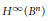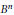Hostname: page-component-546b4f848f-sw5dq Total loading time: 0 Render date: 2023-05-30T19:00:37.572Z Has data issue: false Feature Flags: { "useRatesEcommerce": true } hasContentIssue false

# An Infinite Dimensional Vector Space of Universal Functions for H∞ of the Ball

Published online by Cambridge University Press:  20 November 2018

## Abstract

HTML view is not available for this content. However, as you have access to this content, a full PDF is available via the ‘Save PDF’ action button.

We show that there exists a closed infinite dimensional subspace of${{H}^{\infty }}\left( {{B}^{n}} \right)$ such that every function of norm one is universal for some sequence of automorphisms of${{B}^{n}}$.

## Keywords

Type
Research Article
Information
Canadian Mathematical Bulletin , 01 June 2007 , pp. 172 - 181

## References

 Axler, S. and Gorkin, P., Sequences in the maximal ideal space of H . Proc. Amer. Math. Soc. 108(1990), no. 3, 731740.Google Scholar
 Bayart, F., Universal inner functions on the ball. To appear, Canad. Math. Bull.Google Scholar
 Bernal-González, L. and Montes-Rodríguez, A., Non-finite-dimensional closed vector spaces of universal functions for composition operators. J. Approx. Theory 82(1995), no. 3, 375391.CrossRefGoogle Scholar
 Birkhoff, G. D., Démonstration d’un théorème élémentaire sur les fonctions entiéres. C. R. Acad. Sci. Paris 189(1929), 473475.Google Scholar
 Chee, P. S., Universal functions in several complex variables. J. Austral. Math. Soc. Ser. A 28(1979), no. 2, 189196.CrossRefGoogle Scholar
 Gauthier, P. M. and Xiao, J., The existence of universal inner functions on the unit ball of Cn . Canad. Math. Bull. 49(2005), no. 3, 409413.CrossRefGoogle Scholar
 Gorkin, P. and Mortini, R. Asymptotic interpolating sequences in uniform algebras. J. London Math. Soc. (2) 67(2003), no. 2, 481498.CrossRefGoogle Scholar
 Gorkin, P. and Mortini, R., Universal Blaschke products. Math. Proc. Cambridge Philos. Soc. 136(2004), no. 1, 175184.CrossRefGoogle Scholar
 Gorkin, P. and Mortini, R., Universal singular inner functions. Canad. Math. Bull. 47(2004), no. 1, 1721.CrossRefGoogle Scholar
 Grosse-Erdmann, K.-G., Universal families and hypercyclic operators. Bull. Amer. Math. Soc. (N.S.) 36(1999), no. 3, 345381.CrossRefGoogle Scholar
 Heins, M., A universal Blaschke product. Archiv Math. 6(1954), 4144.CrossRefGoogle Scholar
 Hosokawa, T., Izuchi, K., and Zheng, D., Isolated points and essential components of composition operators on H . Proc. Amer. Math. Soc. 130(2002), no. 6, 17651773 (electronic).CrossRefGoogle Scholar
 Montes-Rodríguez, A., Vector spaces of universal functions. In: Complex methods in approximation theory. Monogr. Cienc. Tecnol. 2, Universidad Almería, Almería, 1997, pp. 113116,.Google Scholar
 Montes-Rodríguez, A., A note on Birkhoff open sets. Complex Variables Theory Appl. 30(1996), no. 3, 193198.CrossRefGoogle Scholar
 Mortini, R., Infinite dimensional universal subspaces generated by Blaschke products. Proc. Amer. Math. Soc. 135(2007), no. 6, 17951801.CrossRefGoogle Scholar
 Rudin, W., Function theory in the unit ball of Cn. Grundlehren der Mathematischen Wissenschaften 241. Springer-Verlag, New York, 1980.CrossRefGoogle Scholar
 Seidel, W. and Walsh, J. L., On approximation by euclidean and non-euclidean translations of an analytic function. Bull. Amer. Math. Soc. 47(1941), 916920.CrossRefGoogle Scholar